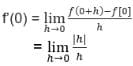# Test: Mean Value Theorem

## 5 Questions MCQ Test Mathematics (Maths) Class 12 | Test: Mean Value Theorem

Description
Attempt Test: Mean Value Theorem | 5 questions in 10 minutes | Mock test for JEE preparation | Free important questions MCQ to study Mathematics (Maths) Class 12 for JEE Exam | Download free PDF with solutions
QUESTION: 1

### Geometrically the Mean Value theorem ensures that there is at least one point on the curve f(x) , whose abscissa lies in (a, b) at which the tangent is

Solution:

GMVT states that for a given arc between two endpoints, there is at least one point at which the tangent to the arc is parallel to the secant through its endpoints.

QUESTION: 2

### When Rolle’s Theorem is verified for f(x) on [a, b] then there exists c such that​

Solution:

B) c ∈ (a, b) such that f'(c) = 0.
Statement for Rolle’s Theorem :
Suppose that a function f(x) is continuous on the closed interval [a,b] and differentiable on the open interval (a,b). Then if f(a)=f(b), then there exists at least one point c in the open interval (a,b) for which f′(c)=0.

QUESTION: 3

### If the function f (x) = x2– 8x + 12 satisfies the condition of Rolle’s Theorem on (2, 6), find the value of c such that f ‘(c) = 0​

Solution:

f (x) = x2 - 8x + 12
Function satisfies the condition of Rolle's theorem for (2,6).
We need to find c for which f’(c) = 0
f’(x) = 2x – 8
f’(c) = 2c – 8 = 0
c = 4

QUESTION: 4

The value of c for which Lagrange’s theorem f(x) = |x| in the interval [-1, 1] is​

Solution:

For LMVT to be valid on a function in an interval, the function should be continuous and differentiable on the interval
Here,
f(x) = |x| , Interval : [-1,1]For h>0,
f’(0) = 1
For h<0,
f’(0) = -1
So, the LHL and RHL are unequal hence f(x) is not differentiable at x=0.
In [-1,1], there does not exist any value of c for which LMVT is valid.

QUESTION: 5

To verify Rolle’s Theorem which one is essential?

Solution:

Rolle’s Theorem:
If a real-valued function f is continuous on a proper closed interval [a, b], differentiable on the open interval (a, b), and f (a) = f (b), then there exists at least one c in the open interval (a, b) such that
f’(c) = 0Use Code STAYHOME200 and get INR 200 additional OFF Use Coupon Code